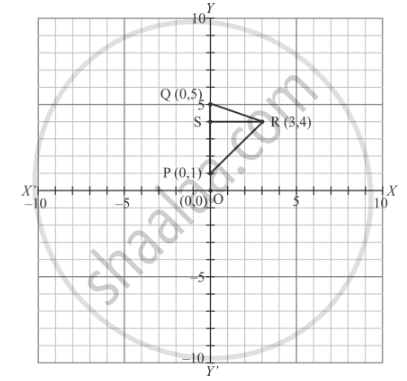# The Area of the Triangle Formed by the Points P (0, 1), Q (0, 5) and R (3, 4) is - Mathematics

MCQ

The area of the triangle formed by the points P (0, 1), Q (0, 5) and R (3, 4) is

• 16 sq. units

• 8 sq. units

• 4 sq. units

• 6 sq. units

#### Solution

Given that the points P (0, 1), Q (0, 5) and R (3, 4) is form a triangle.

We are asked to find the area of the triangle ΔPQR which is shown in the figure.Given that

OP = 1

and OQ = 5

Hence

PQ = OQ - OP

= 5-1

= 4

and RS = 3

By using formula,

"PQR "= 1/2 xx "PQ "xx "RS"

 = 1/2 xx 4 xx 3

 = 2 xx 3

 =6 " sq . units"

Concept: Coordinate Geometry
Is there an error in this question or solution?

#### APPEARS IN

RD Sharma Mathematics for Class 9
Chapter 8 Co-ordinate Geometry
Exercise 8.2 | Q 15 | Page 7

Share